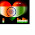Sunday, October 14, 2012

Time and Distance problems

1. Average Speed=Total distance/Total time

• When they are more than one average speed then in order to find the average speeds of all here are some conditions
1. When time is constant Average speed is Average of all speeds.
2. When distance is constant then average speed is Harmonic mean of all average speeds.

For e.g: Two speeds s1,s2 Average speed=2/((1/s1)+(1/s2))

A train of length ‘l’ speed ‘s’ crossing a pole(man) of negligible length then time taken by train to train is

T=l/s
2    A train of length ‘l’ and speed ‘s’ crossing a platform of length ‘lp’, then time taken by train to cross platform is
T=
(l+lp)/s

Relative speed Problems:

In solving Relative speed problems there arises two cases
Case 1: When two objects of speeds s1, s2 are moving in the same direction then relative speed is difference of their speeds (i.e., s1~s2)
Case 2: When two objects of speeds s1, s2 are moving in the opposite direction then relative speed is sum of their speeds (i.e., s1+s2)

To remember more things constant repetition is a way. The mind only remembers a quarter of what you hear.

1.